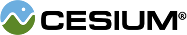#QuarticRealPolynomial

#### QuarticRealPolynomial() Core/QuarticRealPolynomial.js 19

Defines functions for 4th order polynomial functions of one variable with only real coefficients.

### Methods

#### staticCesium.QuarticRealPolynomial.computeDiscriminant(a, b, c, d, e) → Number Core/QuarticRealPolynomial.js 31

Provides the discriminant of the quartic equation from the supplied coefficients.
Name Type Description
`a` Number The coefficient of the 4th order monomial.
`b` Number The coefficient of the 3rd order monomial.
`c` Number The coefficient of the 2nd order monomial.
`d` Number The coefficient of the 1st order monomial.
`e` Number The coefficient of the 0th order monomial.
##### Returns:
The value of the discriminant.

#### staticCesium.QuarticRealPolynomial.computeRealRoots(a, b, c, d, e) → Array.<Number> Core/QuarticRealPolynomial.js 257

Provides the real valued roots of the quartic polynomial with the provided coefficients.
Name Type Description
`a` Number The coefficient of the 4th order monomial.
`b` Number The coefficient of the 3rd order monomial.
`c` Number The coefficient of the 2nd order monomial.
`d` Number The coefficient of the 1st order monomial.
`e` Number The coefficient of the 0th order monomial.
##### Returns:
The real valued roots.